Test: Introduction to Electrical & Electronics Measurements

# Test: Introduction to Electrical & Electronics Measurements

Test Description

## 10 Questions MCQ Test GATE Electrical Engineering (EE) 2023 Mock Test Series | Test: Introduction to Electrical & Electronics Measurements

Test: Introduction to Electrical & Electronics Measurements for Electrical Engineering (EE) 2023 is part of GATE Electrical Engineering (EE) 2023 Mock Test Series preparation. The Test: Introduction to Electrical & Electronics Measurements questions and answers have been prepared according to the Electrical Engineering (EE) exam syllabus.The Test: Introduction to Electrical & Electronics Measurements MCQs are made for Electrical Engineering (EE) 2023 Exam. Find important definitions, questions, notes, meanings, examples, exercises, MCQs and online tests for Test: Introduction to Electrical & Electronics Measurements below.
Solutions of Test: Introduction to Electrical & Electronics Measurements questions in English are available as part of our GATE Electrical Engineering (EE) 2023 Mock Test Series for Electrical Engineering (EE) & Test: Introduction to Electrical & Electronics Measurements solutions in Hindi for GATE Electrical Engineering (EE) 2023 Mock Test Series course. Download more important topics, notes, lectures and mock test series for Electrical Engineering (EE) Exam by signing up for free. Attempt Test: Introduction to Electrical & Electronics Measurements | 10 questions in 30 minutes | Mock test for Electrical Engineering (EE) preparation | Free important questions MCQ to study GATE Electrical Engineering (EE) 2023 Mock Test Series for Electrical Engineering (EE) Exam | Download free PDF with solutions
 1 Crore+ students have signed up on EduRev. Have you?
Test: Introduction to Electrical & Electronics Measurements - Question 1

### in present day measurement systems

Detailed Solution for Test: Introduction to Electrical & Electronics Measurements - Question 1

Measurement by direct methods are not always possible, feasible and practicable. In most of the cases direct method of measurements are inaccurate because they involve human factors. Moreover, they are less sensitive. Due to these reasons use of direct method of measurement is limited while indirect methods are commonly used

Test: Introduction to Electrical & Electronics Measurements - Question 2

### The use of electronic instruments is becoming more extensive because they have

Test: Introduction to Electrical & Electronics Measurements - Question 3

### A thermometer is calibrated 150°C to 200°C. The accuracy is specified within ±0.25 percent of instrument span. The maximum static error is

Detailed Solution for Test: Introduction to Electrical & Electronics Measurements - Question 3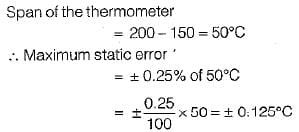Test: Introduction to Electrical & Electronics Measurements - Question 4

A set of independent current measurements were recorded as 10.03, 10.10, 10.08 and 10.11 A, The average range of error is

Detailed Solution for Test: Introduction to Electrical & Electronics Measurements - Question 4

Average value of current is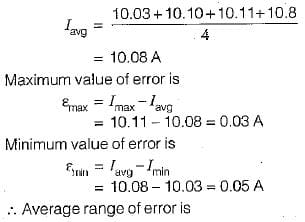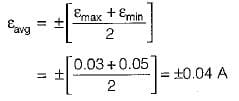Test: Introduction to Electrical & Electronics Measurements - Question 5

Two resistors R1, and R2 are connected in series with R1 = 28.7 Ω and R2 = 3.624 Ω. The total resistance to the appropriate number of significant figures can be written as

Detailed Solution for Test: Introduction to Electrical & Electronics Measurements - Question 5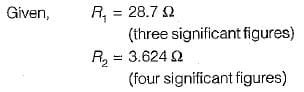For series connection,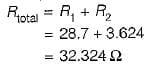Since one of the resistance (i.e. R1) is accurate only to three significant figures, therefore the result of R total has to be reduced to three significant figures. Hence, total resistance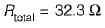Test: Introduction to Electrical & Electronics Measurements - Question 6

A Wheatstone bridge requires a change of 8 a in the unknown arm of the bridge to produce a change in deflection of 3 mm of the galvanometer. The deflection factor (scale factor) is

Detailed Solution for Test: Introduction to Electrical & Electronics Measurements - Question 6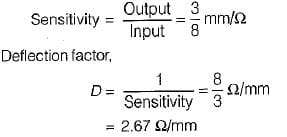Test: Introduction to Electrical & Electronics Measurements - Question 7

A moving coil voltmeter has a uniform scale with 100 divisions, the full scale reading is 200 V and 1/10 of a scale division can be estimated with a fair degree of certainty.The resolution of the instrument in volt is

Detailed Solution for Test: Introduction to Electrical & Electronics Measurements - Question 7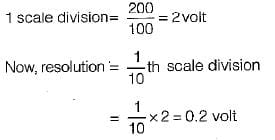Test: Introduction to Electrical & Electronics Measurements - Question 8

A multimeter having a sensitivity of 2 kΩ/V is used for the measurement of voltage across a circuit having an output resistance of 10 kΩ. The open circuit voltage of the circuit is 6 volts. The percentage error in multimeter reading when it is set to 10 volt is given by

Detailed Solution for Test: Introduction to Electrical & Electronics Measurements - Question 8

The circuit diagram for the measurement of voltage using multimeter is shown below: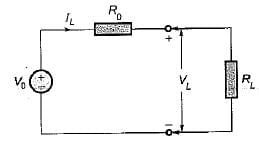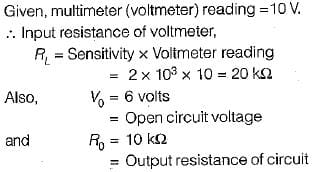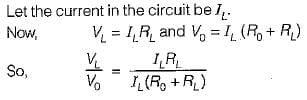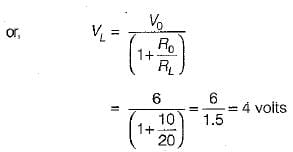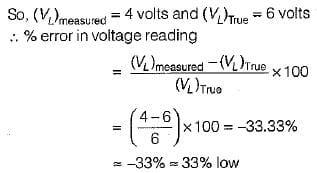Test: Introduction to Electrical & Electronics Measurements - Question 9

A 50 V range voltmeter is connected across the terminals X and Y of the circuit shown in figure below. The voltage across the terminals are measured both under open circuit and loaded conditions.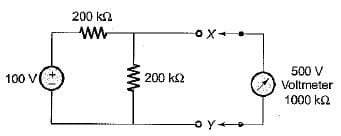The accuracy in the measurement of voltage across the terminals X and Y in percent is

Detailed Solution for Test: Introduction to Electrical & Electronics Measurements - Question 9

The given circuit can be reduced to its Thevenin’s equivalent circuit as shown below.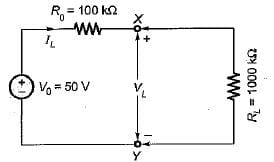True value of voltage across terminals X-Y is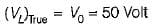Measured value of voltage across terminals X-Y is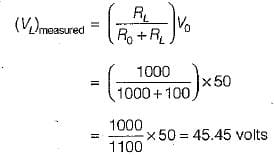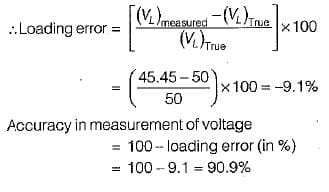Test: Introduction to Electrical & Electronics Measurements - Question 10

The number of significant figures in the two resistors having resistances 4 x 106 kΩ and 0.345 kΩ are respectively

## GATE Electrical Engineering (EE) 2023 Mock Test Series

22 docs|274 tests
Information about Test: Introduction to Electrical & Electronics Measurements Page
In this test you can find the Exam questions for Test: Introduction to Electrical & Electronics Measurements solved & explained in the simplest way possible. Besides giving Questions and answers for Test: Introduction to Electrical & Electronics Measurements, EduRev gives you an ample number of Online tests for practice

## GATE Electrical Engineering (EE) 2023 Mock Test Series

22 docs|274 tests(Scan QR code)